Olympiad Test Level 2: Numbers - 1

# Olympiad Test Level 2: Numbers - 1

Test Description

## 10 Questions MCQ Test Math Olympiad for Class 2 | Olympiad Test Level 2: Numbers - 1

Olympiad Test Level 2: Numbers - 1 for Class 2 2022 is part of Math Olympiad for Class 2 preparation. The Olympiad Test Level 2: Numbers - 1 questions and answers have been prepared according to the Class 2 exam syllabus.The Olympiad Test Level 2: Numbers - 1 MCQs are made for Class 2 2022 Exam. Find important definitions, questions, notes, meanings, examples, exercises, MCQs and online tests for Olympiad Test Level 2: Numbers - 1 below.
Solutions of Olympiad Test Level 2: Numbers - 1 questions in English are available as part of our Math Olympiad for Class 2 for Class 2 & Olympiad Test Level 2: Numbers - 1 solutions in Hindi for Math Olympiad for Class 2 course. Download more important topics, notes, lectures and mock test series for Class 2 Exam by signing up for free. Attempt Olympiad Test Level 2: Numbers - 1 | 10 questions in 20 minutes | Mock test for Class 2 preparation | Free important questions MCQ to study Math Olympiad for Class 2 for Class 2 Exam | Download free PDF with solutions
 1 Crore+ students have signed up on EduRev. Have you?
Olympiad Test Level 2: Numbers - 1 - Question 1

### How many tens make 120?

Detailed Solution for Olympiad Test Level 2: Numbers - 1 - Question 1

12 tens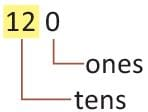Olympiad Test Level 2: Numbers - 1 - Question 2

### Which one of the following is true for 36?

Detailed Solution for Olympiad Test Level 2: Numbers - 1 - Question 2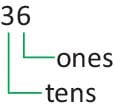Olympiad Test Level 2: Numbers - 1 - Question 3

### Match the following: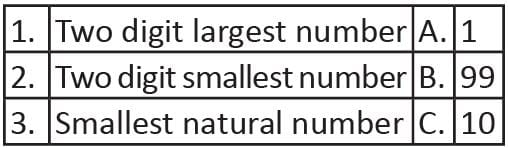Olympiad Test Level 2: Numbers - 1 - Question 4

What is the tens’ digit is the number 124 ?

Detailed Solution for Olympiad Test Level 2: Numbers - 1 - Question 4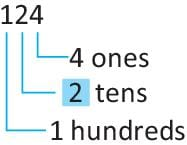Olympiad Test Level 2: Numbers - 1 - Question 5

Write 658 in words

Olympiad Test Level 2: Numbers - 1 - Question 6

Select the INCORRECT match.

Detailed Solution for Olympiad Test Level 2: Numbers - 1 - Question 6

15 tens more than 68 ones
= 150 + 68
= 218
= Two hundred and eighteen

Olympiad Test Level 2: Numbers - 1 - Question 7

Which one of the following is the smallest two digit number?

Detailed Solution for Olympiad Test Level 2: Numbers - 1 - Question 7

10 is the smallest two digit number.

Olympiad Test Level 2: Numbers - 1 - Question 8

The digit at the place of hundreds in the number 712 is:

Detailed Solution for Olympiad Test Level 2: Numbers - 1 - Question 8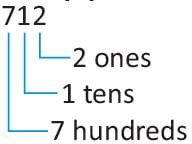Olympiad Test Level 2: Numbers - 1 - Question 9

Which of the following statements is correct for 234?

Detailed Solution for Olympiad Test Level 2: Numbers - 1 - Question 9

Number 234 has 2 hundreds, 3 tens, 4 ones.

Olympiad Test Level 2: Numbers - 1 - Question 10

Direction: Write in the expanded notation for following number.

468

Detailed Solution for Olympiad Test Level 2: Numbers - 1 - Question 10

468 = 400 + 60 + 8

## Math Olympiad for Class 2

72 tests
 Use Code STAYHOME200 and get INR 200 additional OFF Use Coupon Code
Information about Olympiad Test Level 2: Numbers - 1 Page
In this test you can find the Exam questions for Olympiad Test Level 2: Numbers - 1 solved & explained in the simplest way possible. Besides giving Questions and answers for Olympiad Test Level 2: Numbers - 1, EduRev gives you an ample number of Online tests for practice

72 tests